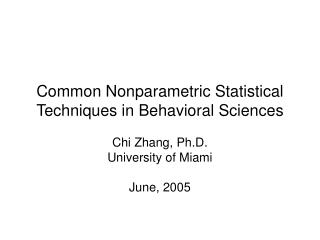DownloadDownload PresentationCommon Nonparametric Statistical Techniques in Behavioral Sciences

# Common Nonparametric Statistical Techniques in Behavioral Sciences

Download Presentation## Common Nonparametric Statistical Techniques in Behavioral Sciences

- - - - - - - - - - - - - - - - - - - - - - - - - - - E N D - - - - - - - - - - - - - - - - - - - - - - - - - - -
##### Presentation Transcript

1. Common Nonparametric Statistical Techniques in Behavioral Sciences Chi Zhang, Ph.D. University of Miami June, 2005

2. Objectives • Assumptions for nonparametric statistics • Scales of measurement • Advantages and disadvantages of nonparametric statistics • Nonparametric tests for the single-sample case • Nonparametric tests for two related samples • Nonparametric tests for two independent samples • The case of k related samples • The case of k independent samples

3. Assumptions about Parametric Statistics • A normally distributed population • Equal variances among the population • The observation must be independent • The variables must be measured at interval or ratio scale

4. Assumptions about Nonparametric Statistics • Nonparametric (distribution free) techniques make no assumptions bout the population • The fewer the assumptions, the more general are the conclusions • The more powerful tests are those that have the strongest or most extensive assumptions

5. Advantages of Nonparametric Statistical Tests • May be the only test when the sample size is small • Require fewer assumptions • The only choice when the measurement scales are nominal or ordinal (e.g. using categories, rankings, medians)

6. Disadvantages of Nonparametric Statistical Tests • They are less powerful • They are unfamiliar to many researchers and editors

7. Scales of Measurement • Nominal or categorical (e.g. gender, nationality) • Ordinal (e.g. ranking, ratings) • Interval (e.g. temperature) • Ratio (e.g. age, distance, weight)

8. The Chi-square Goodness of Fit (Single-sample Case) • It assesses the degree of correspondence between the observed and expected observations in each category • Measurement scale: nominal or categorical • Small expected frequencies (when df = 1, freq (exp) => 5; when df > 1, 20%+ freq (exp) => 5)

9. The Kolmogorov-Smirnov One-sample Test (Single-sample Case) • It tests the goodness of fit for variable which are measured on at least ordinal scale • It involves specifying the cumulative frequency distribution which would occur given the theoretical distribution ( e.g. normal distribution) and comparing that with the observed cumulative frequency distribution

10. The NcNemar Test(Two related samples) • It is particularly applicable to “before and after” designs in which each subject is used as its own control • The measurements are made on either a nominal or ordinal scale

11. The Sign Test(Two related samples) • For research in which quantitative measurement is impossible or infeasible • It is possible to determine, for each pair of observations, which is the “greater” • The only assumption is that the variable has a continuous distribution

12. The Wilcoxon Signed Rank Test(Two related samples) • All the observations must be measured at ordinal scale • Ranking the differences observed for the various matched pairs • Power-efficiency is about 95% of that of paired t-test

13. The Chi-square Test for Two Independent Samples • Suitable for nominal or stronger data • Determining whether the two samples are from populations that differ in any respect at all (e.g. location, dispersion, skewness)

14. The Kolmogorov-Smirnov Two Sample Tests • Ordinal or stronger data • It tests whether two independent samples have been drawn from populations with the same distribution • The test is concerned with the agreement between two cumulative distributions

15. The Man-Whitney U Test(two independent samples) • It tests whether two samples represent populations that differ in central tendency • Variables measured at least at ordinal scale • One of the most powerful of the nonparametric tests • A useful alternative to t-test

16. The Case of k Related Samples • The Friedman two-way ANOVA by ranks is appropriate when the measurements of the variables are at least ordinal • The Friedman two-way ANOVA by ranks tests the probability that the k related samples could have come from the same population with respect the mean rankings. • The Cochran Q test (nominal data)

17. The Case of k Independent Samples • The Kruskal-Wallis one-way ANOVA by ranks tests tha null hypothesis that the k samples come from the same population or from identical populations with the same median • The Kruskal-Wallis one-way ANOVA by ranks requires at ordinal measurement of the variable • The Chi-square test (nominal data) and the Median test (ordinal data)

18. Choice of Statistical Tests

19. Reference • Siegel, Sidney & Castellan, N. John, Jr (1988). Nonparametric statistics for the behavioral sciences (2nd edition). New York: McGraw-Hill, 1988.